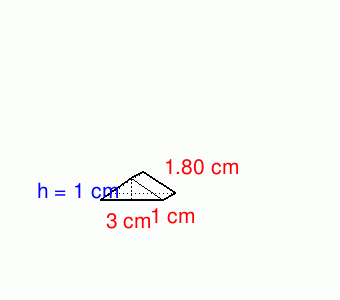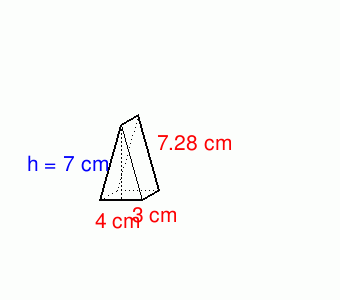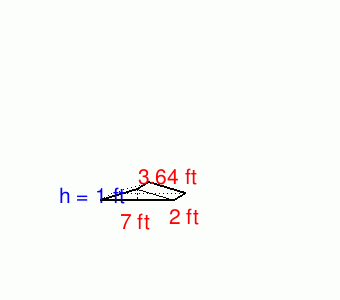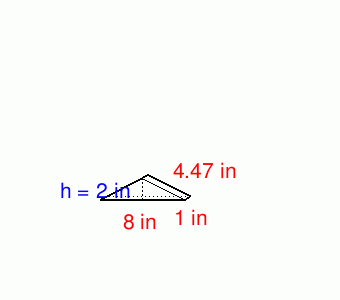# Math Practice Topic: Triangular Prisms

 Description: Find the volumes of triangular prisms. Adaptive Learning Progression: More problems, higher difficulty. Start using MathScore for free

## Sample Levels (out of 6)

Find the volume of the triangular prisms given. Answer with proper units.

 1volume =

Find the volume of the triangular prisms given. Answer with proper units.

 1volume = 2volume = 3volume =